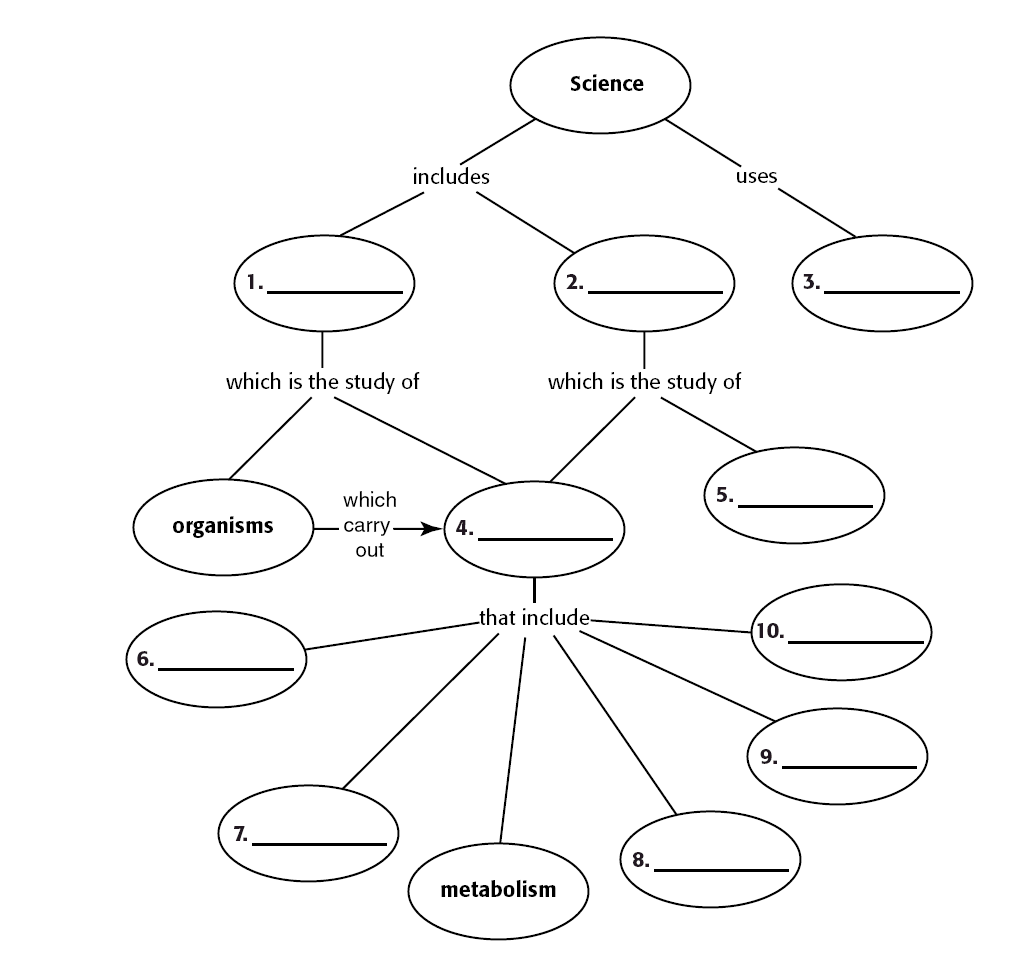# Graphing Practice

Graphing PracticeIntroduction

• Graphing is an important procedure used by scientists to display the data that is collected during a controlled experiment
• Line graphs must be constructed correctly to accurately portray the data collected
• Many times the wrong construction of a graph detracts from the acceptance of an individual’s hypothesis
• A graph contains five major parts:
a. Title
b. The independent variable
c. The dependent variable
d. The scales for each variable
e. A legend
• The title: depicts what the graph is about. By reading the title, the reader should get an idea about the graph. It should be a concise statement placed above the graph.
• The Independent Variable: is the variable that can be controlled by the experimenter. It usually includes time (dates, minutes, hours), depth (feet, meters), temperature (Celsius). This variable is placed on the X axis (horizontal axis).
• The Dependent Variable: is the variable that is directly affected by the independent variable. It is the result of what happens because of the independent variable. Example: How many oxygen bubbles are produced by a plant located five meters below the surface of the water? The oxygen bubbles are dependent on the depth of the water. This variable is placed on the Y-axis or vertical axis.
• The Scales for each Variable: In constructing a graph one needs to know where to plot the points representing the data. In order to do this a scale must be employed to include all the data points. This must also take up a conservative amount of space. It is not suggested to have a run on scale making the graph too hard to manage. The scales should start with 0 and climb based on intervals such as: multiples of 2, 5, 10, 20, 25, 50, or 100. The scale of numbers will be dictated by your data values.
• The Legend: is a short descriptive narrative concerning the graph’s data. It should be short and concise and placed under the graph.
• The Mean for a group of variables: To determine the mean for a group of variables, divide the sum of the variables by the total number of variables to get an average.
• The median for a group of variables: To determine median or “middle” for an even number of values, put the values in ascending order and take the average of the two middle values.    e.g.    2, 3, 4, 5, 9, 10     Add 4+5 (2 middle values) and divide by 2 to get 4.5
• The mode for a group of variables: The mode for a group of values is the number that occurs most frequently.     e.g.   2, 5,  8, 2,  6,  11    The number 2 is the mode because it occurred most often (twice)

Procedure 1:
Using the following data, answer the questions below and then construct a line graph.

 Depth in meters Number of Bubbles / minute Plant A Number of Bubbles / minute Plant B 2 29 21 5 36 27 10 45 40 16 32 50 25 20 34 30 10 20

1. What is the dependent variable and why?

2. What is the independent variable and why?

3. What title would you give the graph? .

4. What are the mean, median, and mode of all 3 columns of data?

a). Depth :                      Mean____________Median__________Mode________

b). Bubble Plant A.:        Mean ____________Median_________Mode________

c). Bubbles Plant B:        Mean ____________Median_________Mode________

Graph Title: _________________________________________________________Legend: ______________________________________________________________

Procedure 2:
Diabetes is a disease affecting the insulin producing glands of the pancreas. If there is not enough insulin being produced by these cells, the amount of glucose in the blood will remain high. A blood glucose level above 140 for an extended period of time is not considered normal. This disease, if not brought under control, can lead to severe complications and even death.

Answer the following questions concerning the data below and then graph it.

 Time After Eating hours Glucose mg /dL of Blood Person A Glucose mg /dL of Blood Person B 0.5 170 180 1 155 195 1.5 140 230 2 135 245 2.5 140 235 3 135 225 4 130 200

1. What is the dependent variable and why?

2. What is the independent variable and why?

3. What title would you give the graph?

4. Which, if any, of the above individuals (A or B) has diabetes?

5. What data do you have to support your hypothesis?

6. If the time period were extended to 6 hours, what would the expected blood glucose level for Person B?

Title: ________________________________________________________________Legend: ______________________________________________________________

Summary:
1. What conclusions can be determined from the data in graph 1?

2. What conclusions can be determined from the data in graph 2?

3. Can the data in each of these graphs be used to construct other types of graphs?

4. If so, what other graph types can be constructed?